We think you are located in South Africa . Is this correct?

• Yes, I reside in South Africa
• Change country/curriculum

We use this information to present the correct curriculum and to personalise content to better meet the needs of our users.## 4.5 Word problems

4.5 word problems (ema3d).

To solve word problems we need to write a set of equations that represent the problem mathematically. The solution of the equations is then the solution to the problem.

## Problem solving strategy (EMA3F)

What are we asked to solve for?

Assign a variable to the unknown quantity, for example, $$x$$.

Translate the words into algebraic expressions by rewriting the given information in terms of the variable.

Set up an equation or system of equations to solve for the variable.

Solve the equation algebraically using substitution.

Check the solution.

The following video shows two examples of working with word problems.

Video: 2FDX

## Worked example 11: Solving word problems

A shop sells bicycles and tricycles. In total there are $$\text{7}$$ cycles (cycles include both bicycles and tricycles) and $$\text{19}$$ wheels. Determine how many of each there are, if a bicycle has two wheels and a tricycle has three wheels.

## Assign variables to the unknown quantities

Let $$b$$ be the number of bicycles and let $$t$$ be the number of tricycles.

## Set up the equations

Rearrange equation $$\left(1\right)$$ and substitute into equation $$\left(2\right)$$, calculate the number of tricycles $$t$$, write the final answer.

There are $$\text{5}$$ tricycles and $$\text{2}$$ bicycles.

## Worked example 12: Solving word problems

Bongani and Jane are friends. Bongani takes Jane's maths test paper and will not tell her what her mark is. He knows that Jane dislikes word problems so he decides to tease her. Bongani says: “I have $$\text{2}$$ marks more than you do and the sum of both our marks is equal to $$\text{14}$$. What are our marks?”

We have two unknown quantities, Bongani's mark and Jane's mark. Let Bongani's mark be $$b$$ and Jane's mark be $$j$$.

## Set up a system of equations

Bongani has $$\text{2}$$ more marks than Jane.

Both marks add up to $$\text{14}$$.

## Use equation $$\left(1\right)$$ to express $$b$$ in terms of $$j$$

Substitute into equation $$\left(2\right)$$, rearrange and solve for $$j$$, substitute the value for $$j$$ back into equation $$\left(1\right)$$ and solve for $$b$$, check that the solution satisfies both original equations.

Bongani got $$\text{8}$$ for his test and Jane got $$\text{6}$$.

## Worked example 13: Solving word problems

A fruitshake costs $$\text{R}\,\text{2,00}$$ more than a chocolate milkshake. If $$\text{3}$$ fruitshakes and $$\text{5}$$ chocolate milkshakes cost $$\text{R}\,\text{78,00}$$, determine the individual prices.

Let the price of a chocolate milkshake be $$x$$ and let the price of a fruitshake be $$y$$.

## Substitute equation $$\left(1\right)$$ into $$\left(2\right)$$

Rearrange and solve for $$x$$, substitute the value of $$x$$ back into equation $$\left(1\right)$$ and solve for $$y$$, write final answer.

One chocolate milkshake costs $$\text{R}\,\text{9,00}$$ and one fruitshake costs $$\text{R}\,\text{11,00}$$.

## Worked example 14: Solving word problems

The product of two consecutive negative integers is $$\text{1 122}$$. Find the two integers.

Let the first integer be $$n$$ and let the second integer be $$n + 1$$

## Set up an equation

Expand and solve for $$n$$, find the sign of the integers.

It is given that both integers must be negative.

The two consecutive negative integers are $$-\text{34}$$ and $$-\text{33}$$.

Two jets are flying towards each other from airports that are $$\text{1 200}$$ $$\text{km}$$ apart. One jet is flying at $$\text{250}$$ $$\text{km·h^{-1}}$$ and the other jet at $$\text{350}$$ $$\text{km·h^{-1}}$$. If they took off at the same time, how long will it take for the jets to pass each other?

Let distance $$d_{1} = \text{1 200} - x\text{ km}$$ and distance $$d_{2} = x\text{ km}$$.

Speed $$s_{1}= \text{250}\text{ km·h^{-1}}$$ and speed $$s_{2}= \text{350}\text{ km·h^{-1}}$$.

Time is found by dividing distance by speed.

When the jets pass each other:

Now we know the distance travelled by the second jet when it passes the first jet, we can find the time:

It will take take the jets 2 hours to pass each other.

Two boats are moving towards each other from harbours that are $$\text{144}$$ $$\text{km}$$ apart. One boat is moving at $$\text{63}$$ $$\text{km·h^{-1}}$$ and the other boat at $$\text{81}$$ $$\text{km·h^{-1}}$$. If both boats started their journey at the same time, how long will they take to pass each other?

Notice that the sum of the distances for the two boats must be equal to the total distance when the boats meet: $$d_{1} + d_{2} = d_{\text{total}} \longrightarrow d_{1} + d_{2} = \text{144}\text{ km}$$.

This question is about distances, speeds, and times. The equation connecting these values is $\text{speed } = \frac{\text{distance }}{\text{time}} \quad \text{- or -} \quad \text{distance } = \text{speed } \times \text{time}$

You want to know the amount of time needed for the boats to meet - let the time taken be $$t$$. Then you can write an expression for the distance each of the boats travels: \begin{align*} \text{For boat 1:} \quad d_{1} &= s_{1} t \\ &= \text{63}t \\ \text{For boat 2:} \quad d_{2} &= s_{2} t \\ &= \text{81}t \end{align*}

Now we can substitute the two expressions for the distances into the expression for the total distance:

The boats will meet after $$\text{1}$$ hour.

Zwelibanzi and Jessica are friends. Zwelibanzi takes Jessica's civil technology test paper and will not tell her what her mark is. He knows that Jessica dislikes word problems so he decides to tease her. Zwelibanzi says: “I have $$\text{12}$$ marks more than you do and the sum of both our marks is equal to $$\text{148}$$. What are our marks?”

Let Zwelibanzi's mark be $$z$$ and let Jessica's mark be $$j$$. Then \begin{align*} z &= j+\text{12} \\ z+j &= \text{148} \end{align*}

Substitute the first equation into the second equation and solve: \begin{align*} z+j &= \text{148} \\ (j+\text{12})+j &= \text{148} \\ 2j &= 148 - \text{12}\\ \therefore j &= \frac{\text{136}}{\text{2}}\\ &= \text{68} \end{align*}

Substituting this value back into the first equation gives: \begin{align*} z &= j+\text{12} \\ &= \text{68}+\text{12} \\ &= \text{80} \end{align*} Zwelibanzi achieved $$\text{80}$$ marks and Jessica achieved $$\text{68}$$ marks.

Kadesh bought $$\text{20}$$ shirts at a total cost of $$\text{R}\,\text{980}$$. If the large shirts cost $$\text{R}\,\text{50}$$ and the small shirts cost $$\text{R}\,\text{40}$$, how many of each size did he buy?

Let $$x$$ be the number of large shirts and $$20 − x$$ the number of small shirts.

Next we note the following:

• He bought $$x$$ large shirts for $$\text{R}\,\text{50}$$
• He bought $$20 - x$$ small shirts for $$\text{R}\,\text{40}$$
• He spent $$\text{R}\,\text{980}$$ in total

We can represent the cost as:

Therefore Kadesh buys $$\text{18}$$ large shirts and $$\text{2}$$ small shirts.

The diagonal of a rectangle is $$\text{25}$$ $$\text{cm}$$ more than its width. The length of the rectangle is $$\text{17}$$ $$\text{cm}$$ more than its width. What are the dimensions of the rectangle?

Let length $$= l$$, width $$= w$$ and diagonal $$= d$$. $$\therefore d = w + 25$$ and $$l = w + 17$$.By the theorem of Pythagoras:

The width must be positive, therefore: width $$w = \text{28}\text{ cm}$$ length $$l = (w + 17) = \text{45}\text{ cm}$$ and diagonal $$d = (w + 25) = \text{53}\text{ cm}$$.

The sum of $$\text{27}$$ and $$\text{12}$$ is equal to $$\text{73}$$ more than an unknown number. Find the unknown number.

Let the unknown number $$= x$$.

The unknown number is $$-\text{34}$$.

A group of friends is buying lunch. Here are some facts about their lunch:

• a milkshake costs $$\text{R}\,\text{7}$$ more than a wrap
• the group buys 8 milkshakes and 2 wraps
• the total cost for the lunch is $$\text{R}\,\text{326}$$

Let a milkshake be $$m$$ and a wrap be $$w$$. From the given information we get the following equations:

Substitute the first equation into the second equation and solve for $$w$$:

Substitute the value of $$w$$ into the first equation and solve for $$m$$:

Therefore a milkshake costs $$\text{R}\,\text{34}$$ and a wrap costs $$\text{R}\,\text{27}$$.

The two smaller angles in a right-angled triangle are in the ratio of $$1:2$$. What are the sizes of the two angles?

Let $$x =$$ the smallest angle. Therefore the other angle $$= 2x$$.

We are given the third angle $$=90°$$.

The sizes of the angles are $$30°$$ and $$60°$$.

The length of a rectangle is twice the breadth. If the area is $$\text{128}$$ $$\text{cm^{2}}$$, determine the length and the breadth.

We are given length $$l = 2b$$ and $$A = l \times b = 128$$.

Substitute the first equation into the second equation and solve for $$b$$:

But breadth must be positive, therefore $$b = 8$$.

Substitute this value into the first equation to solve for $$l$$:

Therefore $$b = \text{8}\text{ cm}$$ and $$l = 2b = \text{16}\text{ cm}$$.

If $$\text{4}$$ times a number is increased by $$\text{6}$$, the result is $$\text{15}$$ less than the square of the number. Find the number.

Let the number $$= x$$. The equation that expresses the given information is:

We are not told if the number is positive or negative. Therefore the number is $$\text{7}$$ or $$-\text{3}$$.

The length of a rectangle is $$\text{2}$$ $$\text{cm}$$ more than the width of the rectangle. The perimeter of the rectangle is $$\text{20}$$ $$\text{cm}$$. Find the length and the width of the rectangle.

Let length $$l = x$$, width $$w = x - 2$$ and perimeter $$= p$$.

$$l = \text{6}\text{ cm}$$ and $$w = l - 2 = \text{4}\text{ cm}$$.

Stephen has 1 litre of a mixture containing $$\text{69}\%$$ salt. How much water must Stephen add to make the mixture $$\text{50}\%$$ salt? Write your answer as a fraction of a litre.

The new volume ($$x$$) of mixture must contain $$\text{50}\%$$ salt, therefore:

The volume of the new mixture is $$\text{1,38}$$ litre The amount of water ($$y$$) to be added is:

Therefore $$\text{0,38}$$ litres of water must be added. To write this as a fraction of a litre: $$\text{0,38} = \frac{38}{100} = \frac{19}{50} \text{ litres}$$

Therefore $$\frac{19}{50} \text{ litres}$$ must be added.

The sum of two consecutive odd numbers is $$\text{20}$$ and their difference is $$\text{2}$$. Find the two numbers.

Let the numbers be $$x$$ and $$y$$.

Then the two equations describing the constraints are:

Add the first equation to the second equation:

Substitute into first equation:

Therefore the two numbers are 9 and 11.

The denominator of a fraction is $$\text{1}$$ more than the numerator. The sum of the fraction and its reciprocal is $$\frac{5}{2}$$. Find the fraction.

Let the numerator be $$x$$. So the denominator is $$x + 1$$.

Solve for $$x$$:

From this the fraction could be $$\frac{1}{2}$$ or $$\frac{-2}{-1}$$. For the second solution we can simplify the fraction to $$\text{2}$$ and in this case the denominator is not 1 less than the numerator.

So the fraction is $$\frac{1}{2}$$.

Masindi is $$\text{21}$$ years older than her daughter, Mulivhu. The sum of their ages is $$\text{37}$$. How old is Mulivhu?

Let Mulivhu be $$x$$ years old. So Masindi is $$x + 21$$ years old.

Mulivhu is $$\text{8}$$ years old.

Tshamano is now five times as old as his son Murunwa. Seven years from now, Tshamano will be three times as old as his son. Find their ages now.

Let Murunwa be $$x$$ years old. So Tshamano is $$5x$$ years old.

In $$\text{7}$$ years time Murunwa's age will be $$x + 7$$. Tshamano's age will be $$5x + 7$$.

So Murunwa is 7 years old and Tshamano is 35 years old.

If adding one to three times a number is the same as the number, what is the number equal to?

Let the number be $$x$$. Then:

If a third of the sum of a number and one is equivalent to a fraction whose denominator is the number and numerator is two, what is the number?

Rearrange until we get a trinomial and solve for $$x$$:

A shop owner buys 40 sacks of rice and mealie meal worth $$\text{R}\,\text{5 250}$$ in total. If the rice costs $$\text{R}\,\text{150}$$ per sack and mealie meal costs $$\text{R}\,\text{100}$$ per sack, how many sacks of mealie meal did he buy?

There are 100 bars of blue and green soap in a box. The blue bars weigh $$\text{50}$$ $$\text{g}$$ per bar and the green bars $$\text{40}$$ $$\text{g}$$ per bar. The total mass of the soap in the box is $$\text{4,66}$$ $$\text{kg}$$. How many bars of green soap are in the box?

Lisa has 170 beads. She has blue, red and purple beads each weighing $$\text{13}$$ $$\text{g}$$, $$\text{4}$$ $$\text{g}$$ and $$\text{8}$$ $$\text{g}$$ respectively. If there are twice as many red beads as there are blue beads and all the beads weigh $$\text{1,216}$$ $$\text{kg}$$, how many beads of each type does Lisa have?• Get started with computers
• Learn Microsoft Office
• Apply for a job
• Improve my work skills
• Design nice-looking docs
• Getting Started
• Smartphones & Tablets
• Typing Tutorial
• Online Learning
• Basic Internet Skills
• Online Safety
• Social Media
• Zoom Basics
• Career Planning
• Resume Writing
• Cover Letters
• Job Search and Networking
• Entrepreneurship 101
• Careers without College
• Job Hunt for Today
• 3D Printing
• Freelancing 101
• Personal Finance
• Sharing Economy
• Decision-Making
• Graphic Design
• Photography
• Image Editing
• Learning WordPress
• Language Learning
• Critical Thinking
• For Educators
• Translations
• Staff Picks
• English expand_more expand_less

## Algebra Topics  - Introduction to Word Problems

Algebra topics  -, introduction to word problems, algebra topics introduction to word problems.## Algebra Topics: Introduction to Word Problems

Lesson 9: introduction to word problems.

/en/algebra-topics/solving-equations/content/

## What are word problems?

A word problem is a math problem written out as a short story or scenario. Basically, it describes a realistic problem and asks you to imagine how you would solve it using math. If you've ever taken a math class, you've probably solved a word problem. For instance, does this sound familiar?

Johnny has 12 apples. If he gives four to Susie, how many will he have left?

You could solve this problem by looking at the numbers and figuring out what the problem is asking you to do. In this case, you're supposed to find out how many apples Johnny has left at the end of the problem. By reading the problem, you know Johnny starts out with 12 apples. By the end, he has 4 less because he gave them away. You could write this as:

12 - 4 = 8 , so you know Johnny has 8 apples left.

## Word problems in algebra

If you were able to solve this problem, you should also be able to solve algebra word problems. Yes, they involve more complicated math, but they use the same basic problem-solving skills as simpler word problems.

You can tackle any word problem by following these five steps:

• Read through the problem carefully, and figure out what it's about.
• Represent unknown numbers with variables.
• Translate the rest of the problem into a mathematical expression.
• Solve the problem.

We'll work through an algebra word problem using these steps. Here's a typical problem:

The rate to rent a small moving van is $30 per day, plus$0.50 per mile. Jada rented a van to drive to her new home. It took two days, and the van cost $360. How many miles did she drive? It might seem complicated at first glance, but we already have all of the information we need to solve it. Let's go through it step by step. ## Step 1: Read through the problem carefully. With any problem, start by reading through the problem. As you're reading, consider: • What question is the problem asking? • What information do you already have? Let's take a look at our problem again. What question is the problem asking? In other words, what are you trying to find out? The rate to rent a small moving van is$30 per day, plus $0.50 per mile. Jada rented a van to drive to her new home. It took 2 days, and the van cost$360. How many miles did she drive?

There's only one question here. We're trying to find out how many miles Jada drove . Now we need to locate any information that will help us answer this question.

There are a few important things we know that will help us figure out the total mileage Jada drove:

• The van cost $30 per day. • In addition to paying a daily charge, Jada paid$0.50 per mile.
• The total cost was $360 . ## Step 2: Represent unknown numbers with variables. In algebra, you represent unknown numbers with letters called variables . (To learn more about variables, see our lesson on reading algebraic expressions .) You can use a variable in the place of any amount you don't know. Looking at our problem, do you see a quantity we should represent with a variable? It's often the number we're trying to find out. Since we're trying to find the total number of miles Jada drove, we'll represent that amount with a variable—at least until we know it. We'll use the variable m for miles . Of course, we could use any variable, but m should be easy to remember.## Step 3: Translate the rest of the problem. Let's take another look at the problem, with the facts we'll use to solve it highlighted. The rate to rent a small moving van is$30 per day , plus $0.50 per mile . Jada rented a van to drive to her new home. It took 2 days , and the van cost$360 . How many miles did she drive?

We know the total cost of the van, and we know that it includes a fee for the number of days, plus another fee for the number of miles. It's $30 per day, and$0.50 per mile. A simpler way to say this would be:

$30 per day plus$0.50 per mile is $360. If you look at this sentence and the original problem, you can see that they basically say the same thing: It cost Jada$30 per day and $0.50 per mile, and her total cost was$360 . The shorter version will be easier to translate into a mathematical expression.

Let's start by translating $30 per day . To calculate the cost of something that costs a certain amount per day, you'd multiply the per-day cost by the number of days—in other words, 30 per day could be written as 30 ⋅ days, or 30 times the number of days . (Not sure why you'd translate it this way? Check out our lesson on writing algebraic expressions .)$30 per day and $.50 per mile is$360

$30 ⋅ day +$.50 ⋅ mile = $360 As you can see, there were a few other words we could translate into operators, so and$.50 became + $.50 ,$.50 per mile became $.50 ⋅ mile , and is became = . Next, we'll add in the numbers and variables we already know. We already know the number of days Jada drove, 2 , so we can replace that. We've also already said we'll use m to represent the number of miles, so we can replace that too. We should also take the dollar signs off of the money amounts to make them consistent with the other numbers. 30 ⋅ 2 + .5 ⋅ m = 360 Now we have our expression. All that's left to do is solve it. ## Step 4: Solve the problem. This problem will take a few steps to solve. (If you're not sure how to do the math in this section, you might want to review our lesson on simplifying expressions .) First, let's simplify the expression as much as possible. We can multiply 30 and 2, so let's go ahead and do that. We can also write .5 ⋅ m as 0.5 m . 60 + .5m = 360 Next, we need to do what we can to get the m alone on the left side of the equals sign. Once we do that, we'll know what m is equal to—in other words, it will let us know the number of miles in our word problem. We can start by getting rid of the 60 on the left side by subtracting it from both sides . The only thing left to get rid of is .5 . Since it's being multiplied with m , we'll do the reverse and divide both sides of the equation with it. .5 m / .5 is m and 300 / 0.50 is 600 , so m = 600 . In other words, the answer to our problem is 600 —we now know Jada drove 600 miles. ## Step 5: Check the problem. To make sure we solved the problem correctly, we should check our work. To do this, we can use the answer we just got— 600 —and calculate backward to find another of the quantities in our problem. In other words, if our answer for Jada's distance is correct, we should be able to use it to work backward and find another value, like the total cost. Let's take another look at the problem. According to the problem, the van costs$30 per day and $0.50 per mile. If Jada really did drive 600 miles in 2 days, she could calculate the cost like this:$30 per day and $0.50 per mile 30 ⋅ day + .5 ⋅ mile 30 ⋅ 2 + .5 ⋅ 600 According to our math, the van would cost$360, which is exactly what the problem says. This means our solution was correct. We're done!

While some word problems will be more complicated than others, you can use these basic steps to approach any word problem. On the next page, you can try it for yourself.

Let's practice with a couple more problems. You can solve these problems the same way we solved the first one—just follow the problem-solving steps we covered earlier. For your reference, these steps are:

If you get stuck, you might want to review the problem on page 1. You can also take a look at our lesson on writing algebraic expressions for some tips on translating written words into math.

Try completing this problem on your own. When you're done, move on to the next page to check your answer and see an explanation of the steps.

A single ticket to the fair costs $8. A family pass costs$25 more than half of that. How much does a family pass cost?

Here's another problem to do on your own. As with the last problem, you can find the answer and explanation to this one on the next page.

Flor and Mo both donated money to the same charity. Flor gave three times as much as Mo. Between the two of them, they donated $280. How much money did Mo give? ## Problem 1 Answer Here's Problem 1: A single ticket to the fair costs$8. A family pass costs $25 more than half that. How much does a family pass cost? Answer:$29

Let's solve this problem step by step. We'll solve it the same way we solved the problem on page 1.

## Step 1: Read through the problem carefully

The first in solving any word problem is to find out what question the problem is asking you to solve and identify the information that will help you solve it . Let's look at the problem again. The question is right there in plain sight:

So is the information we'll need to answer the question:

• A single ticket costs $8 . • The family pass costs$25 more than half the price of the single ticket.

## Step 2: Represent the unknown numbers with variables

The unknown number in this problem is the cost of the family pass . We'll represent it with the variable f .

## Step 3: Translate the rest of the problem

Let's look at the problem again. This time, the important facts are highlighted.

A single ticket to the fair costs $8 . A family pass costs$25 more than half that . How much does a family pass cost?

In other words, we could say that the cost of a family pass equals half of $8, plus$25 . To turn this into a problem we can solve, we'll have to translate it into math. Here's how:

• First, replace the cost of a family pass with our variable f .

f equals half of $8 plus$25

• Next, take out the dollar signs and replace words like plus and equals with operators.

f = half of 8 + 25

• Finally, translate the rest of the problem. Half of can be written as 1/2 times , or 1/2 ⋅ :

f = 1/2 ⋅ 8 + 25

## Step 4: Solve the problem

Now all we have to do is solve our problem. Like with any problem, we can solve this one by following the order of operations.

• f is already alone on the left side of the equation, so all we have to do is calculate the right side.
• First, multiply 1/2 by 8 . 1/2 ⋅ 8 is 4 .
• Next, add 4 and 25. 4 + 25 equals 29 .

That's it! f is equal to 29. In other words, the cost of a family pass is $29 . ## Step 5: Check your work Finally, let's check our work by working backward from our answer. In this case, we should be able to correctly calculate the cost of a single ticket by using the cost we calculated for the family pass. Let's look at the original problem again. We calculated that a family pass costs$29. Our problem says the pass costs $25 more than half the cost of a single ticket. In other words, half the cost of a single ticket will be$25 less than $29. • We could translate this into this equation, with s standing for the cost of a single ticket. 1/2s = 29 - 25 • Let's work on the right side first. 29 - 25 is 4 . • To find the value of s , we have to get it alone on the left side of the equation. This means getting rid of 1/2 . To do this, we'll multiply each side by the inverse of 1/2: 2 . According to our math, s = 8 . In other words, if the family pass costs$29, the single ticket will cost $8. Looking at our original problem, that's correct! So now we're sure about the answer to our problem: The cost of a family pass is$29 .

Here's Problem 2:

Answer: $70 Let's go through this problem one step at a time. Start by asking what question the problem is asking you to solve and identifying the information that will help you solve it . What's the question here? To solve the problem, you'll have to find out how much money Mo gave to charity. All the important information you need is in the problem: • The amount Flor donated is three times as much the amount Mo donated • Flor and Mo's donations add up to$280 total

The unknown number we're trying to identify in this problem is Mo's donation . We'll represent it with the variable m .

Here's the problem again. This time, the important facts are highlighted.

Flor and Mo both donated money to the same charity. Flor gave three times as much as Mo . Between the two of them, they donated $280 . How much money did Mo give? The important facts of the problem could also be expressed this way: Mo's donation plus Flor's donation equals$280

Because we know that Flor's donation is three times as much as Mo's donation, we could go even further and say:

Mo's donation plus three times Mo's donation equals $280 We can translate this into a math problem in only a few steps. Here's how: • Because we've already said we'll represent the amount of Mo's donation with the variable m , let's start by replacing Mo's donation with m . m plus three times m equals$280

• Next, we can put in mathematical operators in place of certain words. We'll also take out the dollar sign.

m + three times m = 280

• Finally, let's write three times mathematically. Three times m can also be written as 3 ⋅ m , or just 3 m .

m + 3m = 280

It will only take a few steps to solve this problem.

• To get the correct answer, we'll have to get m alone on one side of the equation.
• To start, let's add m and 3 m . That's 4 m .
• We can get rid of the 4 next to the m by dividing both sides by 4. 4 m / 4 is m , and 280 / 4 is 70 .

We've got our answer: m = 70 . In other words, Mo donated $70 . The answer to our problem is$70 , but we should check just to be sure. Let's look at our problem again.

If our answer is correct, $70 and three times$70 should add up to $280 . • We can write our new equation like this: 70 + 3 ⋅ 70 = 280 • The order of operations calls for us to multiply first. 3 ⋅ 70 is 210. 70 + 210 = 280 • The last step is to add 70 and 210. 70 plus 210 equals 280 . 280 is the combined cost of the tickets in our original problem. Our answer is correct : Mo gave$70 to charity./en/algebra-topics/distance-word-problems/content/• Kindergarten
• Number charts
• Skip Counting
• Place Value
• Number Lines
• Subtraction
• Multiplication
• Word Problems
• Comparing Numbers
• Ordering Numbers
• Odd and Even
• Prime and Composite
• Roman Numerals
• Ordinal Numbers
• In and Out Boxes
• Number System Conversions
• More Number Sense Worksheets
• Size Comparison
• Measuring Length
• Metric Unit Conversion
• Customary Unit Conversion
• Temperature
• More Measurement Worksheets
• Writing Checks
• Profit and Loss
• Simple Interest
• Compound Interest
• Tally Marks
• Mean, Median, Mode, Range
• Mean Absolute Deviation
• Stem-and-leaf Plot
• Box-and-whisker Plot
• Permutation and Combination
• Probability
• Venn Diagram
• More Statistics Worksheets
• Shapes - 2D
• Shapes - 3D
• Lines, Rays and Line Segments
• Points, Lines and Planes
• Transformation
• Ordered Pairs
• Midpoint Formula
• Distance Formula
• Parallel, Perpendicular and Intersecting Lines
• Scale Factor
• Surface Area
• Pythagorean Theorem
• More Geometry Worksheets
• Converting between Fractions and Decimals
• Significant Figures
• Convert between Fractions, Decimals, and Percents
• Proportions
• Direct and Inverse Variation
• Order of Operations
• Squaring Numbers
• Square Roots
• Scientific Notations
• Speed, Distance, and Time
• Absolute Value
• More Pre-Algebra Worksheets
• Translating Algebraic Phrases
• Evaluating Algebraic Expressions
• Simplifying Algebraic Expressions
• Algebraic Identities
• Systems of Equations
• Polynomials
• Inequalities
• Sequence and Series
• Complex Numbers
• More Algebra Worksheets
• Trigonometry
• Math Workbooks
• English Language Arts
• Summer Review Packets
• Social Studies
• Holidays and Events
• Worksheets >
• Algebra >
• Equations >

## Equation Word Problems Worksheets

This compilation of a meticulously drafted equation word problems worksheets is designed to get students to write and solve a variety of one-step, two-step and multi-step equations that involve integers, fractions, and decimals. These worksheets are best suited for students in grade 6 through high school. Click on the 'Free' icons to sample our handouts.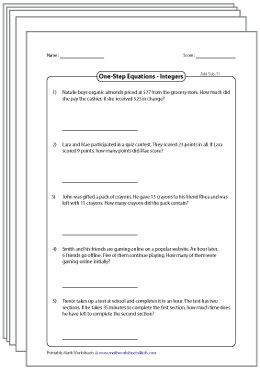One Step Equation Word Problem Worksheets

Read and solve this series of word problems that involve one-step equations. Apply basic operations to find the value of unknowns.

(15 Worksheets)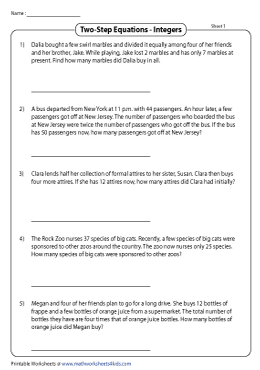Two-Step Equation Word Problems: Integers

Interpret this set of word problems that require two-step operations to solve the equations. Each printable worksheet has five word problems ideal for 6th grade, 7th grade, and 8th grade students.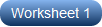Two-Step Equation Word Problems: Fractions and Decimals

Read each word problem and set up the two-step equation. Solve the equation and find the solution. This selection of worksheets includes both fractions and decimals.MCQ - Two-Step Equation Word Problems

Pick the correct two-step equation that best matches word problems presented here. Evaluate the ability of students to solve two-step equations with this array of MCQ worksheets.Multi-Step Equation Word Problems: Integers

Read each multi-step word problem in these high school pdf worksheets and set up the equation. Solve and find the value of the unknown. More than two steps are required to solve the problems.Multi-step equation Word Problems: Fractions and Decimals

Write multi-step equations that involve both fractions and decimals based on the word problems provided here. Validate your responses with our answer keys.Related Worksheets

» One-step Equation

» Two-step Equation

» Multi-step Equation

» Algebraic Identities

Become a Member

Membership Information

What's New?

Printing Help

TestimonialMembers have exclusive facilities to download an individual worksheet, or an entire level.## Word Problems on Linear Equations

Worked-out word problems on linear equations with solutions explained step-by-step in different types of examples.

There are several problems which involve relations among known and unknown numbers and can be put in the form of equations. The equations are generally stated in words and it is for this reason we refer to these problems as word problems. With the help of equations in one variable, we have already practiced equations to solve some real life problems.

Steps involved in solving a linear equation word problem: ● Read the problem carefully and note what is given and what is required and what is given. ● Denote the unknown by the variables as x, y, ……. ● Translate the problem to the language of mathematics or mathematical statements. ● Form the linear equation in one variable using the conditions given in the problems. ● Solve the equation for the unknown. ● Verify to be sure whether the answer satisfies the conditions of the problem.

## Step-by-step application of linear equations to solve practical word problems:

1. The sum of two numbers is 25. One of the numbers exceeds the other by 9. Find the numbers.

Solution: Then the other number = x + 9 Let the number be x.  Sum of two numbers = 25 According to question, x + x + 9 = 25 ⇒ 2x + 9 = 25 ⇒ 2x = 25 - 9 (transposing 9 to the R.H.S changes to -9)  ⇒ 2x = 16 ⇒ 2x/2 = 16/2 (divide by 2 on both the sides)  ⇒ x = 8 Therefore, x + 9 = 8 + 9 = 17 Therefore, the two numbers are 8 and 17.

2.The difference between the two numbers is 48. The ratio of the two numbers is 7:3. What are the two numbers?  Solution:   Let the common ratio be x.  Let the common ratio be x.  Their difference = 48 According to the question,  7x - 3x = 48  ⇒ 4x = 48  ⇒ x = 48/4  ⇒ x = 12 Therefore, 7x = 7 × 12 = 84           3x = 3 × 12 = 36  Therefore, the two numbers are 84 and 36.

3. The length of a rectangle is twice its breadth. If the perimeter is 72 metre, find the length and breadth of the rectangle.  Solution: Let the breadth of the rectangle be x,  Then the length of the rectangle = 2x Perimeter of the rectangle = 72 Therefore, according to the question 2(x + 2x) = 72 ⇒ 2 × 3x = 72 ⇒ 6x = 72  ⇒ x = 72/6 ⇒ x = 12 We know, length of the rectangle = 2x                       = 2 × 12 = 24 Therefore, length of the rectangle is 24 m and breadth of the rectangle is 12 m.

4. Aaron is 5 years younger than Ron. Four years later, Ron will be twice as old as Aaron. Find their present ages.

Solution: Let Ron’s present age be x.  Then Aaron’s present age = x - 5 After 4 years Ron’s age = x + 4, Aaron’s age x - 5 + 4.  According to the question;  Ron will be twice as old as Aaron.  Therefore, x + 4 = 2(x - 5 + 4)  ⇒ x + 4 = 2(x - 1)  ⇒ x + 4 = 2x - 2 ⇒ x + 4 = 2x - 2 ⇒ x - 2x = -2 - 4 ⇒ -x = -6 ⇒ x = 6 Therefore, Aaron’s present age = x - 5 = 6 - 5 = 1 Therefore, present age of Ron = 6 years and present age of Aaron = 1 year.

5. A number is divided into two parts, such that one part is 10 more than the other. If the two parts are in the ratio 5 : 3, find the number and the two parts.  Solution: Let one part of the number be x Then the other part of the number = x + 10 The ratio of the two numbers is 5 : 3 Therefore, (x + 10)/x = 5/3 ⇒ 3(x + 10) = 5x  ⇒ 3x + 30 = 5x ⇒ 30 = 5x - 3x ⇒ 30 = 2x  ⇒ x = 30/2  ⇒ x = 15 Therefore, x + 10 = 15 + 10 = 25 Therefore, the number = 25 + 15 = 40  The two parts are 15 and 25.

More solved examples with detailed explanation on the word problems on linear equations.

6. Robert’s father is 4 times as old as Robert. After 5 years, father will be three times as old as Robert. Find their present ages.  Solution: Let Robert’s age be x years.  Then Robert’s father’s age = 4x After 5 years, Robert’s age = x + 5 Father’s age = 4x + 5 According to the question,  4x + 5 = 3(x + 5)  ⇒ 4x + 5 = 3x + 15  ⇒ 4x - 3x = 15 - 5  ⇒ x = 10 ⇒ 4x = 4 × 10 = 40  Robert’s present age is 10 years and that of his father’s age = 40 years.

7. The sum of two consecutive multiples of 5 is 55. Find these multiples.  Solution: Let the first multiple of 5 be x.  Then the other multiple of 5 will be x + 5 and their sum = 55 Therefore, x + x + 5 = 55 ⇒ 2x + 5 = 55 ⇒ 2x = 55 - 5 ⇒ 2x = 50 ⇒ x = 50/2  ⇒ x = 25  Therefore, the multiples of 5, i.e., x + 5 = 25 + 5 = 30 Therefore, the two consecutive multiples of 5 whose sum is 55 are 25 and 30.

8. The difference in the measures of two complementary angles is 12°. Find the measure of the angles.  Solution: Let the angle be x.  Complement of x = 90 - x Given their difference = 12° Therefore, (90 - x) - x = 12° ⇒ 90 - 2x = 12 ⇒ -2x = 12 - 90 ⇒ -2x = -78 ⇒ 2x/2 = 78/2 ⇒ x = 39 Therefore, 90 - x = 90 - 39 = 51  Therefore, the two complementary angles are 39° and 51°

9. The cost of two tables and three chairs is $705. If the table costs$40 more than the chair, find the cost of the table and the chair.  Solution: The table cost $40 more than the chair. Let us assume the cost of the chair to be x. Then the cost of the table =$ 40 + x The cost of 3 chairs = 3 × x = 3x and the cost of 2 tables 2(40 + x)  Total cost of 2 tables and 3 chairs = $705 Therefore, 2(40 + x) + 3x = 705 80 + 2x + 3x = 705 80 + 5x = 705 5x = 705 - 80 5x = 625/5 x = 125 and 40 + x = 40 + 125 = 165 Therefore, the cost of each chair is$125 and that of each table is $165. 10. If 3/5 ᵗʰ of a number is 4 more than 1/2 the number, then what is the number? Solution: Let the number be x, then 3/5 ᵗʰ of the number = 3x/5 Also, 1/2 of the number = x/2 According to the question, 3/5 ᵗʰ of the number is 4 more than 1/2 of the number. ⇒ 3x/5 - x/2 = 4 ⇒ (6x - 5x)/10 = 4 ⇒ x/10 = 4 ⇒ x = 40 The required number is 40. Try to follow the methods of solving word problems on linear equations and then observe the detailed instruction on the application of equations to solve the problems. ● Equations What is an Equation? What is a Linear Equation? How to Solve Linear Equations? Solving Linear Equations Problems on Linear Equations in One Variable Word Problems on Linear Equations in One Variable Practice Test on Linear Equations Practice Test on Word Problems on Linear Equations ● Equations - Worksheets Worksheet on Linear Equations Worksheet on Word Problems on Linear Equation 7th Grade Math Problems 8th Grade Math Practice From Word Problems on Linear Equations to HOME PAGE ## New! Comments Didn't find what you were looking for? Or want to know more information about Math Only Math . Use this Google Search to find what you need. • Preschool Activities • Kindergarten Math • 1st Grade Math • 2nd Grade Math • 3rd Grade Math • 4th Grade Math • 5th Grade Math • 6th Grade Math • 7th Grade Math • 8th Grade Math • 9th Grade Math • 10th Grade Math • 11 & 12 Grade Math • Concepts of Sets • Probability • Boolean Algebra • Math Coloring Pages • Multiplication Table • Cool Maths Games • Math Flash Cards • Online Math Quiz • Math Puzzles • Binary System • Math Dictionary • Conversion Chart • Homework Sheets • Math Problem Ans • Free Math Answers • Printable Math Sheet • Funny Math Answers • Employment Test • Math Patterns • Link Partners • Privacy Policy## Recent Articles## Math Questions Answers | Solved Math Questions and Answers | Free Math Nov 28, 23 01:32 PM## Addition and Subtraction of Units of Measurement | Metric Units Nov 28, 23 12:31 AM## Measuring Capacity | Standard Unit of Capacity | Litre | Millilitres Nov 27, 23 11:10 PM© and ™ math-only-math.com. All Rights Reserved. 2010 - 2023.#### IMAGES 1. #21 Word Problems Involving Multi-Step Equations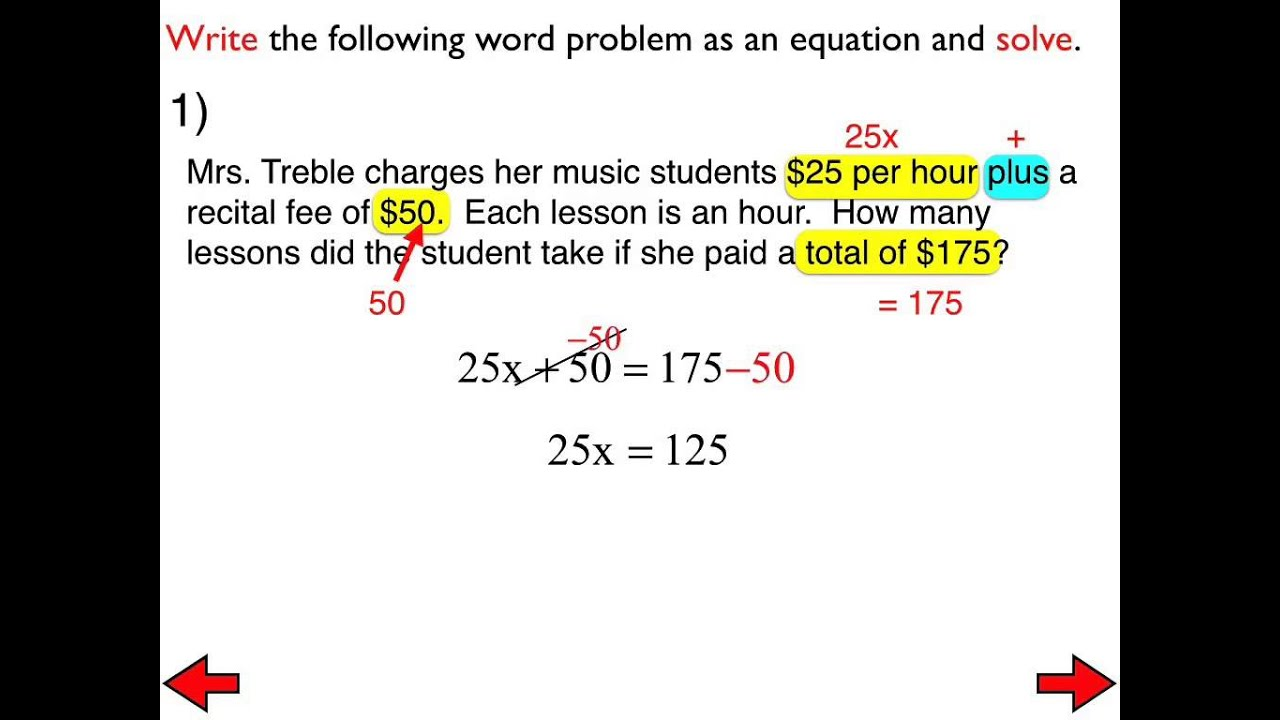2. 040 Solving Equations Word Problems Worksheets Multi Step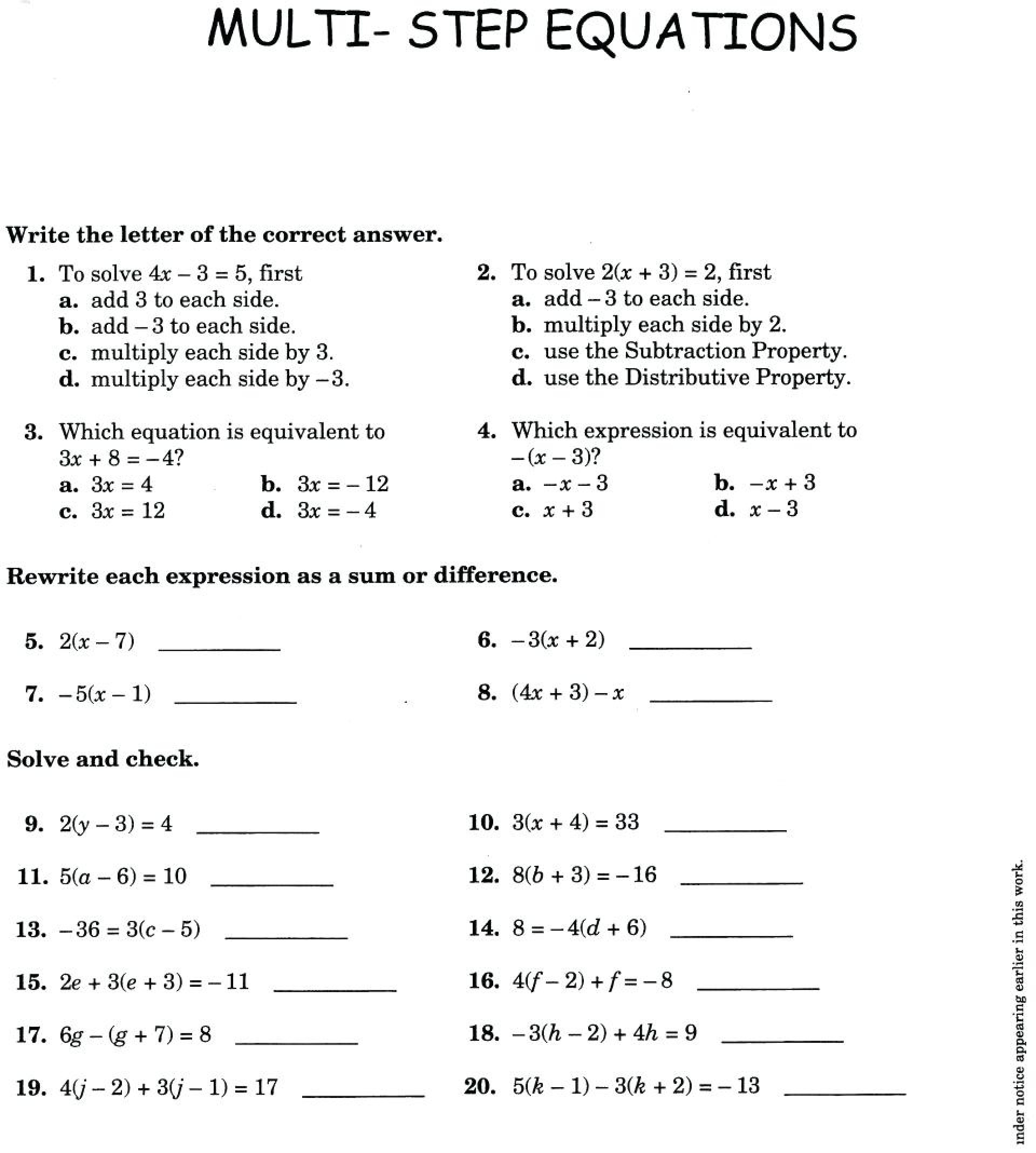3. Linear equation word problems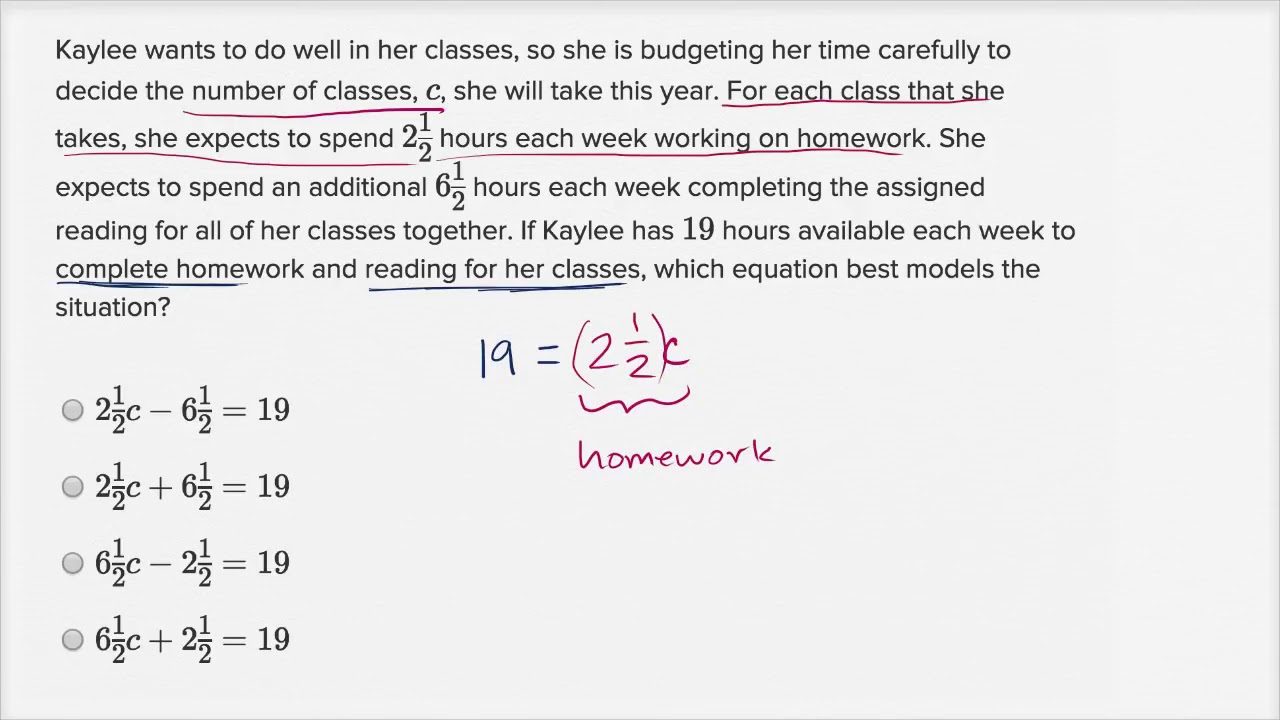4. Linear Equations Word Problems Part 1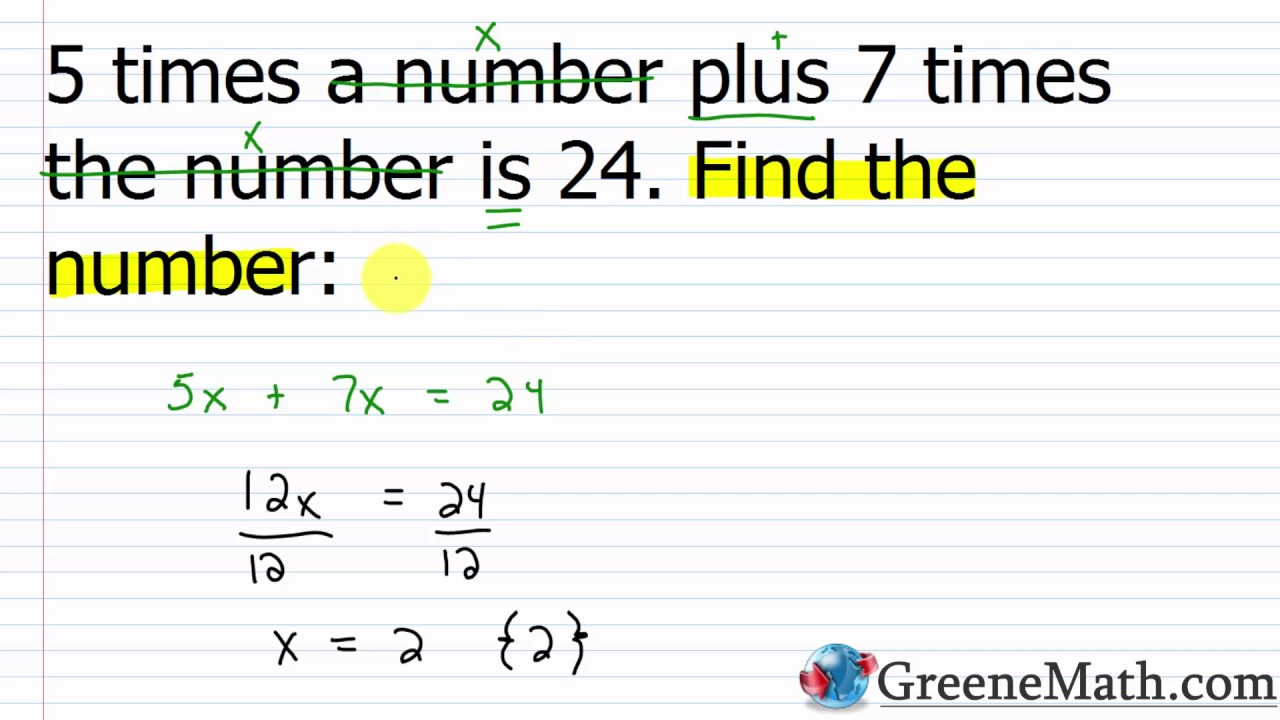5. Equations Word Problems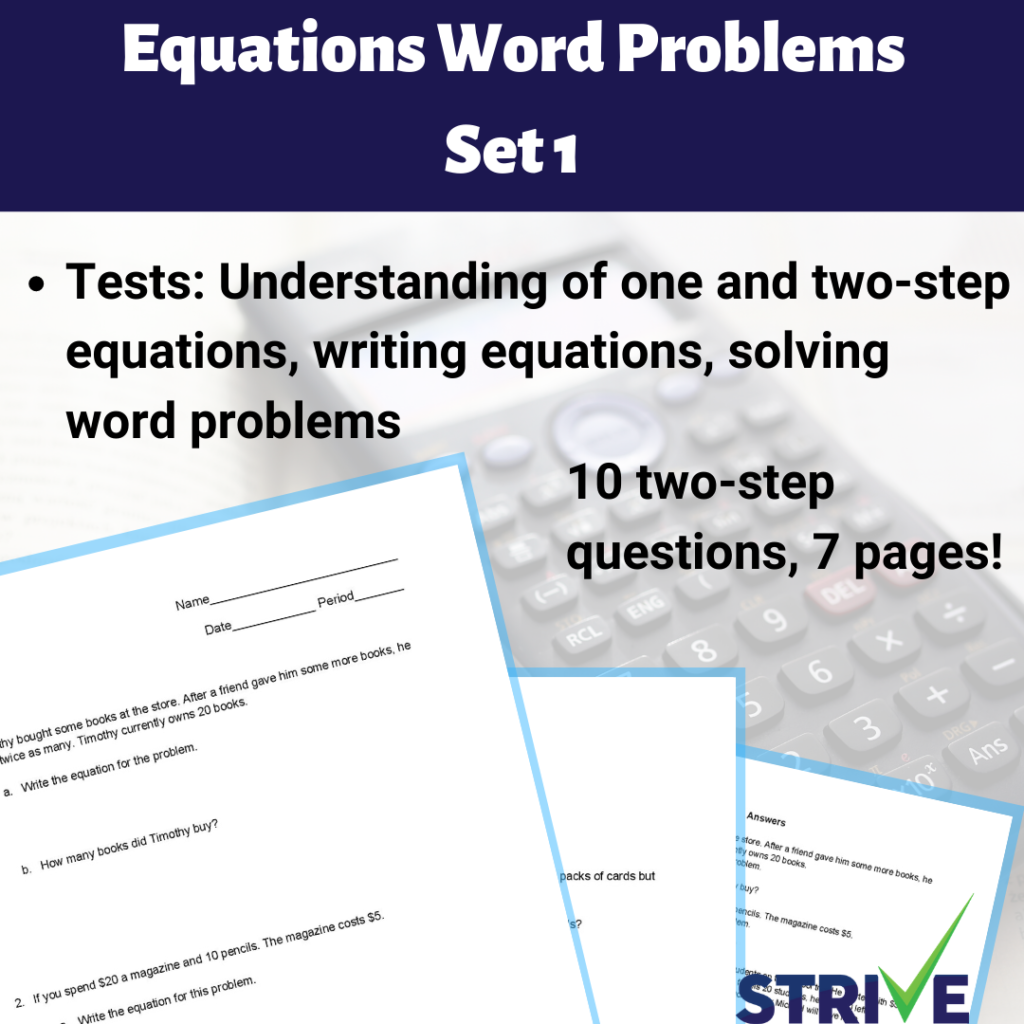6. 3 Variable System Of Equations Word Problems Worksheet#### VIDEO 1. Question 11 From the Equations and Word Problems Test 2. Word Problems Systems of Equations 3. Question 9 From the Equations and Word Problems Test 4. MATH LESSON 28: LINEAR EQUATIONS AND WORD PROBLEMS 5. Question 5 From the Equations and Word Problems Test 6. Question 6 From the Equations and Word Problems Test #### COMMENTS 1. What Is the Equation for Combustion in Words? In words, the equation for combustion, in most cases, is a hydrocarbon plus oxygen equals carbon dioxide plus water plus heat. Other cases involve burning hydrogen and oxygen without carbon and reactions that create carbon monoxide. 2. What Is the Word Equation for Neutralization? The word equation for neutralization is acid + base = salt + water. The acid neutralizes the base, and hence, this reaction is called a neutralization reaction. Neutralization leaves no hydrogen ions in the solution, and the pH of the solut... 3. What Is the Word Equation for Wood Combustion? The word equation for wood combustion is that wood in the presence of oxygen and high heat combusts to produce carbon dioxide, water vapor, heat and ash residue. Wood combustion gives off heat because it is an exothermic reaction. 4. Algebraic word problems Define a variable. · Write an equation using the variable. · Solve the equation. · If the variable is not the answer to the word problem, use the variable to 5. Two-step equations word problems (practice) Problem. Mindy and Troy combined ate 9 ‍ pieces of the wedding cake. Mindy ate 3 ‍ pieces of cake and Troy had 1 4 ‍ of the total cake. 6. Two-step equation word problem: computers (video) We can add 10,000 to both sides. Add 10,000 to both sides, so it's no longer on the right-hand side. And we are left with 10,000. 10,000 is equal to 27x. And 7. Writing Equations from Word Problems Learn how to set up and solve word problems. Although they may seem tricky at first solving word problems can be fun. 8. 4.5 Word problems Mulivhu is $$\text{8}$$ years old. Tshamano is now five times as old 9. Algebra Topics: Introduction to Word Problems /en/algebra-topics/solving-equations/content/. What are word problems? A word problem is a math problem written out as a short story or scenario. 10. Equation Word Problems Worksheets Equation Word Problems Worksheets. This compilation of a meticulously drafted equation word problems worksheets is designed to get students to write and solve a 11. Word Problems on Linear Equations Word Problems on Linear Equations · Then the other number = x + 9. Let the number be x. · 2.The difference between the two numbers is 48. · 3. The length of a 12. Solutions of Word Problems Involving Equations Four places are situated in the order of the letters A. B. C. D. The distance from A to D is 34 miles. The distance from A to B is to the distance from C to D 13. 1.20: Word Problems for Linear Equations 1.20: Word Problems for Linear Equations · 5% · 5%⋅$2.40. · 5%⋅2.40=0.05⋅2.40=0.1200=0.12 · 2.40+0.12=2.52 · $2.52 ·$12 · x · 12⋅3⋅x=180.

14. How to write word problems as equations

Writing the phrase as an algebraic expression · John's age is four less than twice Mary's age. · The next step in solving a word problem is to “